## Pages

Showing posts with label class11-maths. Show all posts
Showing posts with label class11-maths. Show all posts

# National Mathematics Day

National Mathematics Day is observed annually on December 22nd to mark the anniversary of the eminent mathematician Srinivasa Ramanujan's birth.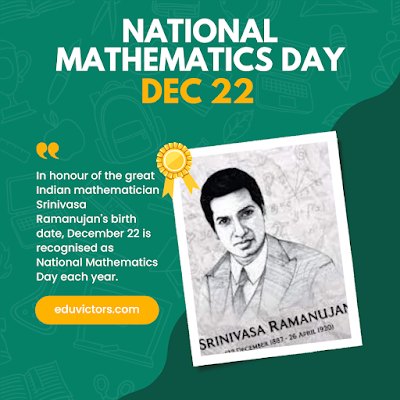# Class 11 - Maths - Trigonometric Functions Notes, Solved Problems and NCERT Exercise 3.2 Solutions

Answer: All angles which are integral multiples of π/2 are called quadrantal angles.

Therefore, for quadrantal angles, we have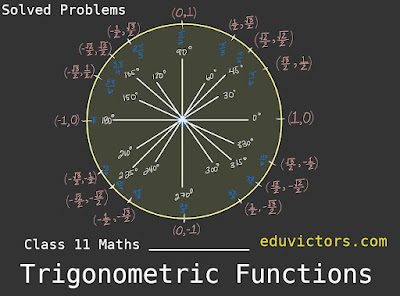# Maths Tip: What are cyclic numbers?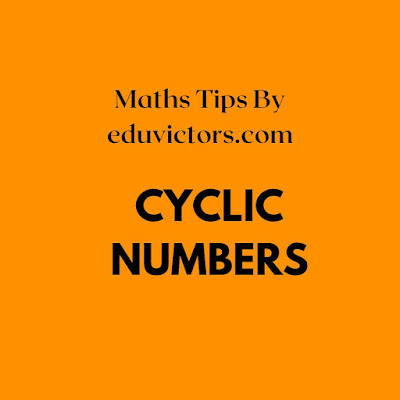# Class 11 - Maths - Trigonometric Functions - Angle on Circular System + NCERT 3.1 Answers. (Part-1)

The phrase "measuring the sides of a triangle" is how the word "trigonometry" is obtained from the Greek words "trigon" and "metron." The field was initially created to address triangle-related geometric issues. The surveyors who mapped out the new territories, the engineers, and other people studied it for navigation. Currently, numerous fields, including the science of seismology, the design of electrical circuits, the description of the condition of an atom, forecasting ocean tide heights, analysing musical tones, and many other things.

You should remember

1. In the sexagesimal system, we measure angles in degrees, minutes and seconds.

1 right angle = 90°

1° = 60′  (minute) and

1′ = 60′′ (second)

2. In the circular measure, we measure angles in radians.

Thus,

πc = 180°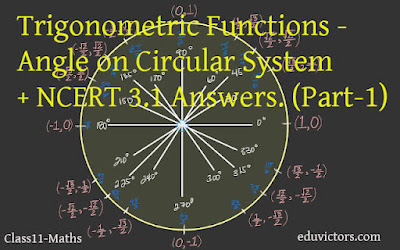# Methods of Differentiation

CBSE Class 11 - Mathematics - Limits and Derivatives Part-11

Working rule for finding the derivative of implicit functions

First method:

Differentiate every term of f (x, y) = 0 with respect to x.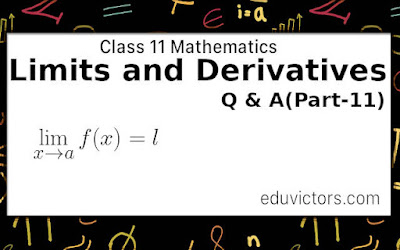# DIFFERENTIATION OF STANDARD FUNCTIONS

CBSE Class 11 - Mathematics - Limits and Derivatives Part-10

You have learnt theorems of differentiation part-9. Let us proceed further. Here is a list of differentiation of some standard functions: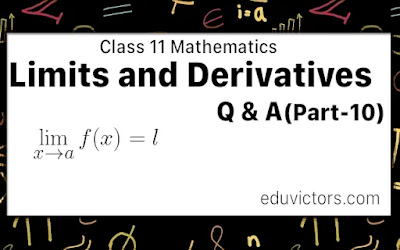# THEOREMS ON DIFFERENTIATION

CBSE Class 11 - Mathematics - Limits and Derivatives Part-9

You have learnt about finding derivatives using the first principle in part-7 and part-8. Let us proceed further. Here is a summary of theorems on differentiation.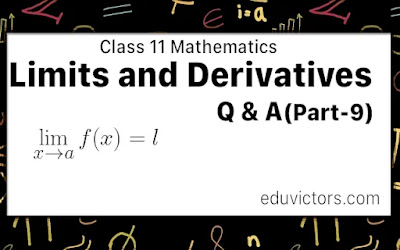# CBSE Class 11 - Mathematics - Limits and Derivatives Part-8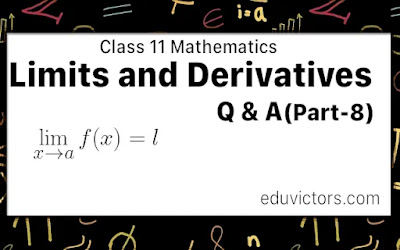Differentiation

In the previous post[Part-7], we learned about some important derivatives using the First Principle.

Let us talk about what differential co-efficient is?

Differential coefficient: Let y = f (x) be a continuous function of a variable quantity x, where x is independent and y. is a dependent variable quantity.# CBSE Class 11 - Mathematics - Limits and Derivatives Part-7

Derivatives Using First Principle

In the previous post[Part-6], we learned about the Derivative of a function at a point. Let us focus on some important derivatives using the First Principle.

Theorem 1: From the first principle, we have# Class 11 - Mathematics - Limits and Derivatives (Part-6) - Derivative of a Function At a Point

In the previous blog post Limits and derivatives Part-5, we learned about the limits. Let us understand the derivative of a function at a point.

Q1: What is the derivative of a function at a point?

Answer: Let y = f(x) is a continuous function. It means the value of y changes as the value of x changes.

At x = a, is a point in its domain of definittion. The derivative of f at a is defines as: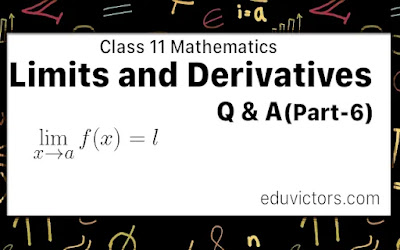# Class 11 & 12 Maths - Probability - Part 1 (Solved Questions)

Let us revisit the basic terminology used in probability.

Q1: Define probability. What can the probability never predict?

Answer: Probability gives us a measure of the likelihood that something will happen. However, probability can never predict the number of times that an occurrence actually happens.

Q2: Define experiment.

Answer: An action or operation resulting in two or more well-defined outcomes.

e.g. tossing a coin, throwing a die, drawing a card from a pack of well-shuffled playing cards etc.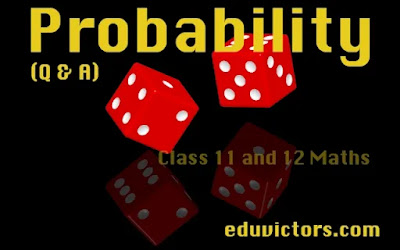# National Mathematics Day

India celebrates National Mathematics Day on December 22 every year. The day marks the birth anniversary of famous mathematician Srinivasa Ramanujan.

*Typo in the graphic new = knew# CBSE Class 11 - Mathematics - Limits and Derivatives Part-5

In the previous blog post Limits and derivatives Part-4, we learned about the  trigonometric limits. Let us solve other problems related to Limits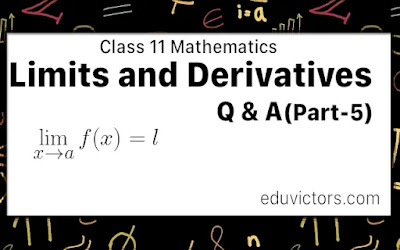# CBSE Class 11 - Mathematics - Limits and Derivatives Part-4- Limits of Trigonometric Functions

In the previous blog post Limits and derivatives Part-3, we learn about the algebra of limits. Now, let us study few theorems before we discuss about trignometric limits.

Theorem 1: Let f(x) and g(x) be two real valued functions with same domain such that f(x) ≤ g(x) for all x.
For some a, if $\lim_{x\rightarrow a} f(x)$ and $\lim_{x\rightarrow a} g(x)$ exist then $\lim_{x\rightarrow a} f(x) \leq \lim_{x\rightarrow a} g(x)$

Theorem 2: Sandwich Theorem or Squeeze Theorem
Let f(x) and g(x) be two real valued functions with same domain such that f(x) ≤ g(x) ≤ h(x) for all x in common domain.
For some a, if $\lim_{x\rightarrow a} f(x) = l = \lim_{x\rightarrow a} h(x)$, then $\lim_{x\rightarrow a} g(x) = l$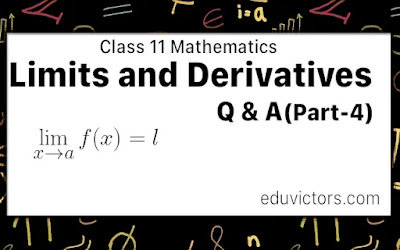# CBSE Class 11 - Mathematics - Limits and Derivatives

Part-3  Algebra of Limits

In the previous post Limits and derivatives Part-2, we get basic ideas of the algebra of limits and also learned about rules and properties of limits.

Let us try to solve few problems:

Q1: Evaluate the given limit $\lim_{r\rightarrow 1} \pi r^2$

Answer: $\lim_{r\rightarrow 1} \pi r^2 = \pi(1)^2 = \pi$# CBSE Class 11 - Mathematics - Limits and Derivatives (Part-2)

In the previous blog post Limits and derivatives Part-1 , we learn

$\lim_{x\rightarrow a} f(x) = l$ and it is called limit of the function f(x)

The two ways x could approach a number an either from left or from right, i.e., all the values of x near a could be less than a or could be greater than a.

In this case the right and left hand limits are different, and hence we say that the limit of f(x) as x tends to zero does not exist (even though the function is defined at 0).# CBSE Class 11 - Mathematics - Limits and Derivatives

Q1: Define Calculus.

Answer: Calculus is that branch of mathematics that mainly deals with the study of change in the value of a function as the points in the domain change.

👉Note: The chapter "Limits and Derivatives" is an introduction to Calculus.

👉Calculus is a Latin word meaning ‘pebble’. Ancient Romans used stones for counting.

Q2: Who are called pioneers of Calculus (who invented Calculus)?

Answer: Issac Newton (1642 - 1727) and G. W. Leibnitz(1646 - 1717).

Both of them were invented independently around the 17th century.

Q3: What is the meaning of 'x tends to a' or x → a?

Answer: When x tends to a (x  → a), x is nearly close to a but never equals to a.

e.g. x → 3 means the value of x maybe 2.99 or 2.999 or 2.999...9 is very close to 3 but not exactly equal to 3. Similarly, x may be 3.01, 3.001, 3.0001... from the right side and gets closer to 3.# CBSE Class 11 Maths Term 1 MCQs For Practice

No. of Questions: 15

Time: 30 minutes

Chapters: Sets, Relations and Functions, Complex Numbers, Sequence and Series, Straight Lines, Limits and Statistics

Q1: The set {1, 2, 3, ...} is _______ set. Fill in the blank.

(a) null

(b) finite

(c) infinite

(d) singleton# Class 11 - Maths - Complex Numbers - Solved Problems - Part 1

Q1: What are imaginary numbers?

Answer: If the square of a given number is negative then such a number is called an imaginary number.

Q2: Name the mathematician who was the first to introduce the symbol i (iota) for square root of -1.

Q3: Evaluate i⁹ + i¹⁹# CBSE Class 11 Maths - Relations - Domain, Range and Co-Domain

RELATION

Let A and B be two nonempty sets. Then, a relation R from A to B is a subset of (A × B).

Thus, R is a relation from A to B ⇔ R ⊆ (A × B).

If (a, b) ∈ R then we say that ‘a is related to b‘ and we write, a R b.

If (a, b) ∉ R then ‘a is not related to b‘ and we write, $a \not \mathrel{R} b$.

Q1: Let A = {-1, 2, 4} and B = {1, 3}. Show A × B as arrow diagram.